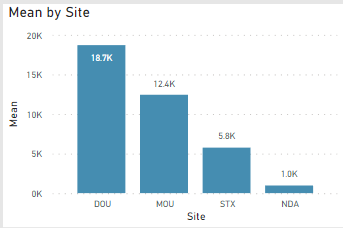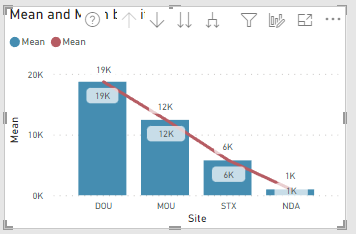cancel
Showing results for
Did you mean:## Creating average as a line in line and bar chart

I have calculated Mean by site for all assets under one site

Mean = DIVIDE('Table'[Hours],Table[F]) , Hours and F are measures in Table

This gives Mean for each siteNow I need to create a line for Average on this chart which would give total Hours for all 4 sites combined/Total F for all 4 sites combined ( to comapre site average with total average)

Using the same Mean as line dosent help1 ACCEPTED SOLUTIONSuper User
``````// NOTE: Measures should never be preceded by
// the table name they sit in. On the other
// hand, columns should always be preceded
// with the name of the table they belong to.

Mean = DIVIDE( [Hours], [F] )

// Assuming that your measures aggregate
// correctly...
[Overall Mean] =
CALCULATE(
[Mean],
ALLSELECTED( T[Site] )
)

// or if the above doesn't work (but it should)...

[Overall Mean] =
DIVIDE(
CALCULATE(
[Hours],
ALLSELECTED( T[Site] )
),
CALCULATE(
[F],
ALLSELECTED( T[Site] )
)
)``````
2 REPLIES 2Super User
``````// NOTE: Measures should never be preceded by
// the table name they sit in. On the other
// hand, columns should always be preceded
// with the name of the table they belong to.

Mean = DIVIDE( [Hours], [F] )

// Assuming that your measures aggregate
// correctly...
[Overall Mean] =
CALCULATE(
[Mean],
ALLSELECTED( T[Site] )
)

// or if the above doesn't work (but it should)...

[Overall Mean] =
DIVIDE(
CALCULATE(
[Hours],
ALLSELECTED( T[Site] )
),
CALCULATE(
[F],
ALLSELECTED( T[Site] )
)
)``````